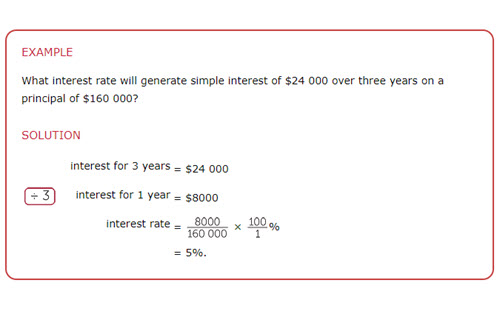Go to website

# The unitary method

This module from the AMSI 'The Improving Mathematics Education in Schools (TIMES) project' is for teachers who wish to consolidate their content knowledge on the topic of coordinate geometry, for example: D47+D50+D47+D50+D47

Year level(s) Year 7, Year 8
Purpose Content knowledge
Keywords ratio, rate, interest, fraction

## Curriculum alignment

Strand and focus Number
Topics Proportion, rates and ratios
AC: Mathematics (V9.0) content descriptions
AC9M7N09
Use mathematical modelling to solve practical problems involving rational numbers and percentages, including financial contexts; formulate problems, choosing representations and efficient calculation strategies, using digital tools as appropriate; interpret and communicate solutions in terms of the situation, justifying choices made about the representation

AC9M8N05

Use mathematical modelling to solve practical problems involving rational numbers and percentages, including financial contexts; formulate problems, choosing efficient calculation strategies and using digital tools where appropriate; interpret and communicate solutions in terms of the situation, reviewing the appropriateness of the model

Interpreting fractions (P8)
Multiplicative strategies (P9)
Proportional thinking (P2, P6)
Understanding money (P8)